# Mazur: Families of rationally connected subvarieties

Introduction

This is joint work with Graber, Harris, and Starr.

Throughout, we let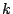be any finite field and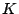a field of transcendence degree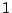over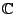. We have two classical results, due to Chevalley-Warning and Tsen, forand, respectively: a hypersurface of low degree has a rational point; a hypersurface of low degree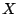is one with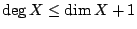.

Inspired by these theorems, one (i.e. Artin) defines a field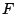to be quasi-algebraically closed if every hypersurface of low degree overhas a-rational point. In view of resent results due to Kollár, Kollár-Miyaoka-Mori, and Graber-Harris-Starr, we ask similar questions not for hypersurfaces but for certain other classes of varieties.

We generalize the notion of hypersurfaces of low degree to rationally connected varieties overwhich are projective and smooth: a varietyis rationally connected if for any two points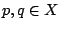, there exists a rational curve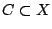with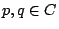. Rationally connected varieties are closed under birational transformation, products, domination (if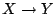is dominant, andis rationally connected, then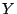is rationally connected), and specialization. This is a much better class of varieties than, say, rational varieties (consider the difficulty in determining which cubic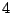-folds are rational).

There is another candidate for a generalization of hypersurfaces of low degree: a varietyoveris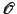-acyclic if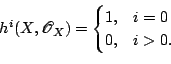Rationally connected varieties are-acyclic.

The only rationally connected curves are rational curves; the only rationally connected surfaces are rational surfaces. However, there are-acyclic surfaces which are not rational, e.g. Enriques surfaces.

Theorem. Ifis a smooth hypersurface over, then it is equivalent forto be of low degree, rationally connected, and-acyclic.

Theorem. [Generalized Chevalley-Warning; Katz] Any-acyclic variety overhas a-rational point.

Over, and given an endomorphism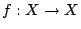, one defines the Lefschetz number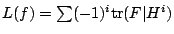; this complex number measures the fixed point locus of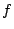. Over a finite field, the Lefschetz number counts the number of fixed points, at least modulo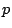; one computes that the Lefschetz number is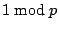.

For the generalization to rationally connected varieties over, a varietyovercan be thought of as a family of rationally connected varieties over a curve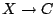. By family we always mean that although the base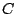might not be proper or smooth, the morphism is proper and generically smooth.

Theorem. [Generalized Tsen; Graber, Harris, Starr] Any rationally connected variety overhas a-rational point.

A converse

Given a family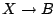, a section is a triangle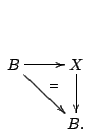We define a pseudo-section to be a triangle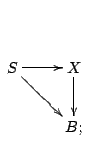where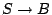is a family of rationally connected varieties. Since a point is rationally connected, a section gives a pseudo-section.

We can rephrase the GHS theorem in this language as follows: If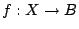is a family with a pseudo-section, then its restriction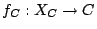to every smooth curve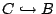has a section.

Theorem. [Weak converse to GHB] Ifis a family such that every restrictionfor every smooth curvehas a section, thenhas a pseudo-section.

This theorem is related to Lefschetz's theorem about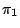as can be seen by restricting attention to finite étale covers.

Applications

This theorem has application to finding varieties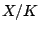with no rational point; in particular:

Corollary. There exists an Enriques surface over somewith no-rational point.

This is completely ineffective; an open question is to find the genus of the curve given by this counterexample.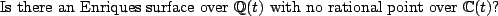Every Enriques surfaces overhas a point over; this is not the case over, so we have distinguished finite fields from function fields of transcendence degreeover. We ask: in the Artin-Lang philosophy, what kinds of varieties are cut out by-acyclic varieties?

We have another corollary:

Corollary. A family of curves of genusover a base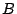has a section if and only if it has a section over every curve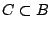.

The corollary is clear: a family of curves of genushas no room for a pseudo-section.

Number Theoretic Applications

Now we consider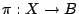a family defined over a number field; we say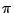is arithmetically surjective if and only if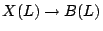is surjective for all finite extensions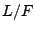.

Ifis a family of curves of genus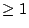, is it the case that arithmetic surjectivity is equivalent to the existence of a section over? This question is unapproachable in full generality.

Instead, let us take a very small fragment of it: letbe a nonempty open subset of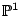over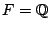,a family of genuscurves, we say it belongs to its Jacobian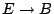. We consider quadratic twist elliptic pencils; given any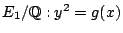, we have the pencil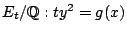. We have the problem: For allbelonging to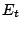, is is true that arithmetic surjectivity holds if and only if a section exists? Work of Skinner-Ono can be used to establish this for all elliptic curves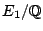.

Back to the main index for Rational and integral points on higher dimensional varieties.